### Eutectoid and Peritectic Systems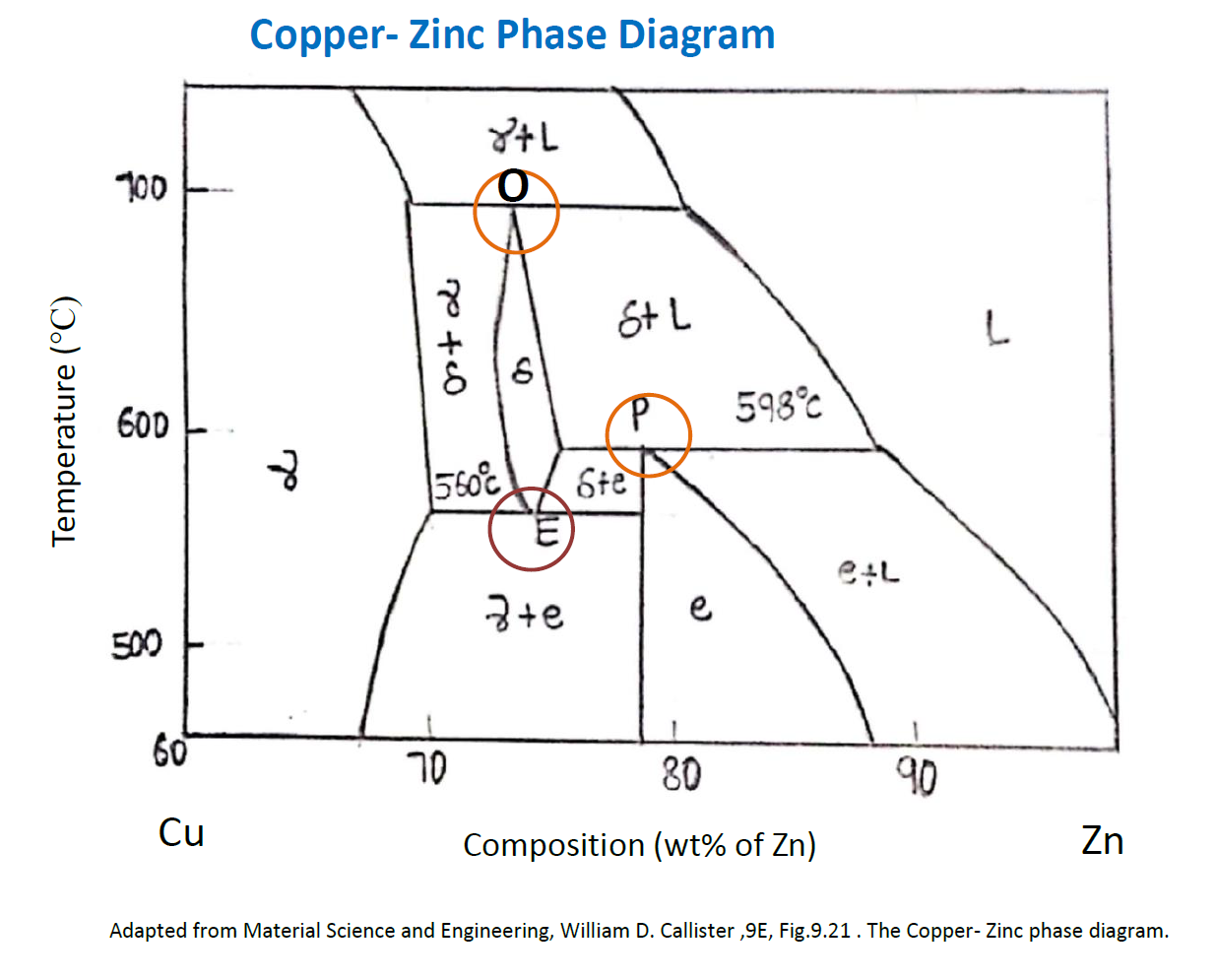An enlarged view of one section of the Cu-Zn system is considered in the above figure to understand the eutectoid and peritectic system.

Eutectoid reaction involves solid to two different solids as indicated at point E in the figure above.Peritectic reaction involves one solid and liquid to other form of solid as indicated at point P and O.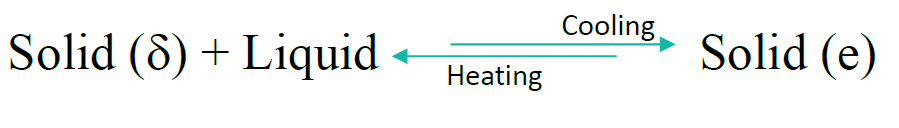The above mentioned phase diagram is of Al-Si system. The eutectic point is at E where liquid is converting to two solids Al+Si at 12.7 wt % Si as shown. Apart from Point E and liquid region, the composition and mass fraction can be determined by drawing tie line. Below 580 °C, we can witness two solid solutions which are partially soluble in each other.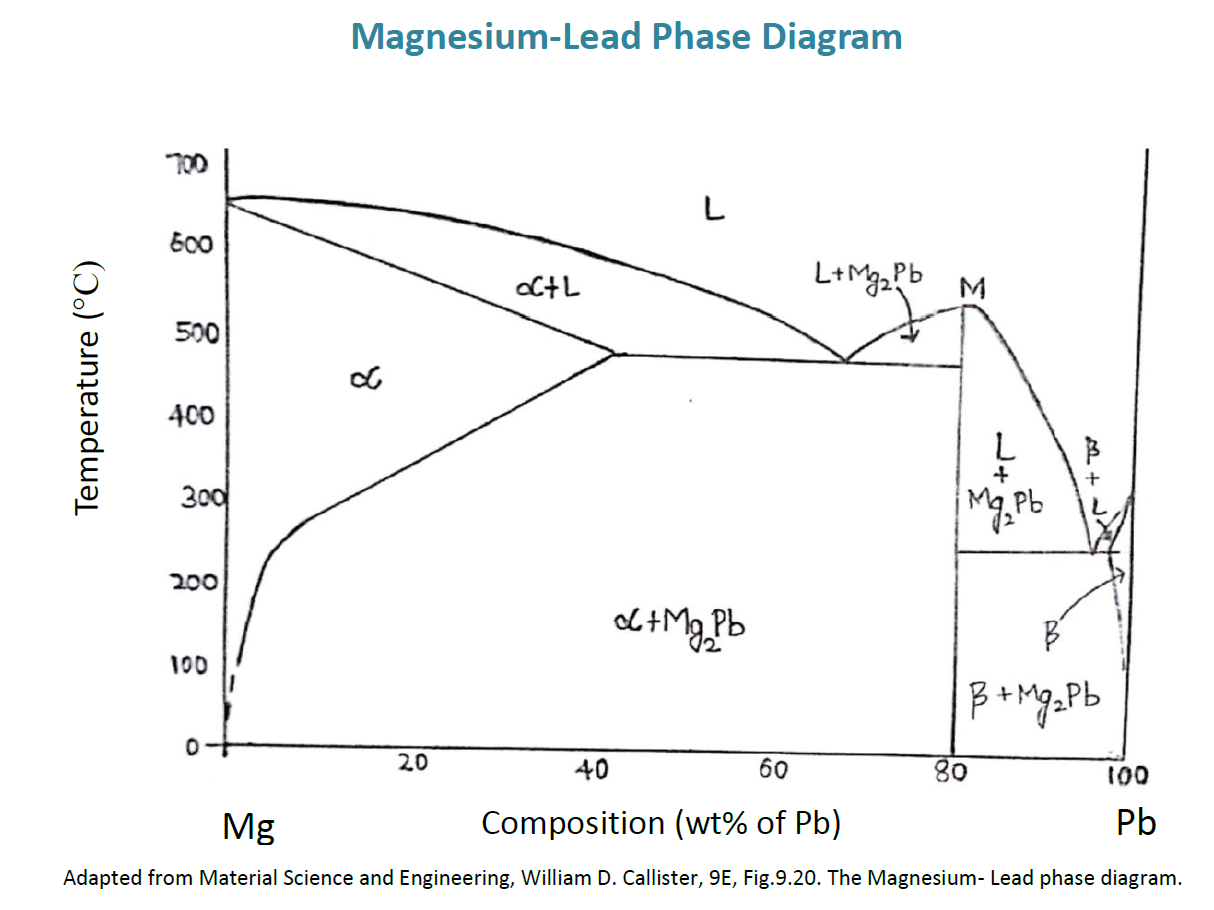For few systems, discrete intermediate compounds instead of solid solutions may be found on the phase diagram, and these compounds contain distinct chemical formulas; in fact for metal–metal systems, they are called intermetallic compounds.The compound Mg2Pb having 19 wt% of Mg and 81 wt% of Pb is shown as a vertical line in the above fig, instead of finite width phase region; Therefore, Mg2Pb can exist by own at this precise composition.
Apart from this, the compound/alloy Mg2Pb melts at nearly 550°C at point M on the above figure. The solubility of Pb in Mg is high, as shown by the relatively large composition span for the a-phase field. But, the solubility of magnesium in lead is limited. This is evident from lead-rich, side of the diagram.

Numerical Problems

1. For a Wt % of 40 Sn – wt% of 60 Pb alloy at the temparature150°C, (a) what are the phases present? (b) What can be the composition(s) of respective phase(s)?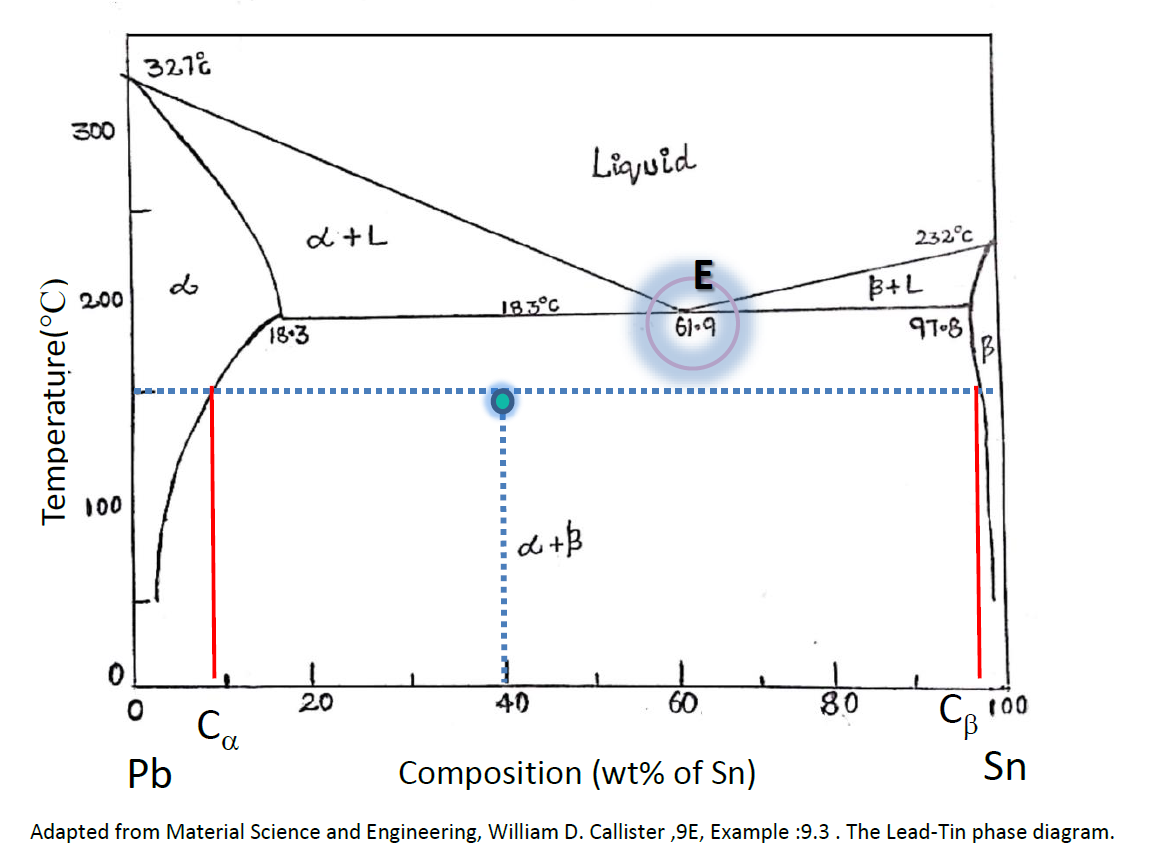1. Indicate this composition – temperature point on the phase diagram. Inasmuch as it’s in the region  + , both  as well as  phases do coexist.

2. As there are two phases, it is necessary to draw a tie line across the  + phase field at the temparature 150°C. The composition of respective ‘a’ phase Corresponds to the tie line meet with the 𝞪 /( 𝞪 + 𝝱) solvus phase boundary—about wt% of 11 Sn– wt% of 89 Pb, mentioned as C𝞪 . This is similar for the ‘b’ phase, which has a composition of approximately wt% of 98 Sn– wt% of 2 Pb (Cᵦ ).

Calculate the relative amount of individual phase present in terms of mass fraction for the lead–tin alloy.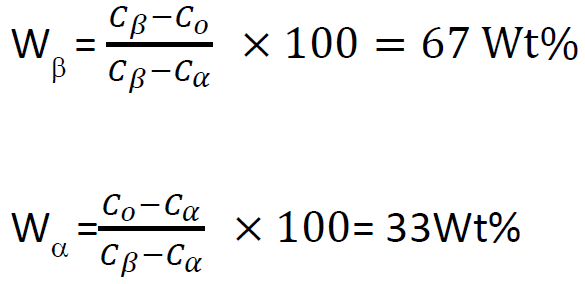1. Find the number of phases and the respective compositions from the diagram, in the binary Cu-Zn alloy, at different temperatures.

2. Given data: Alloy Composition: 74 wt% of Zn – 26 wt % of Cu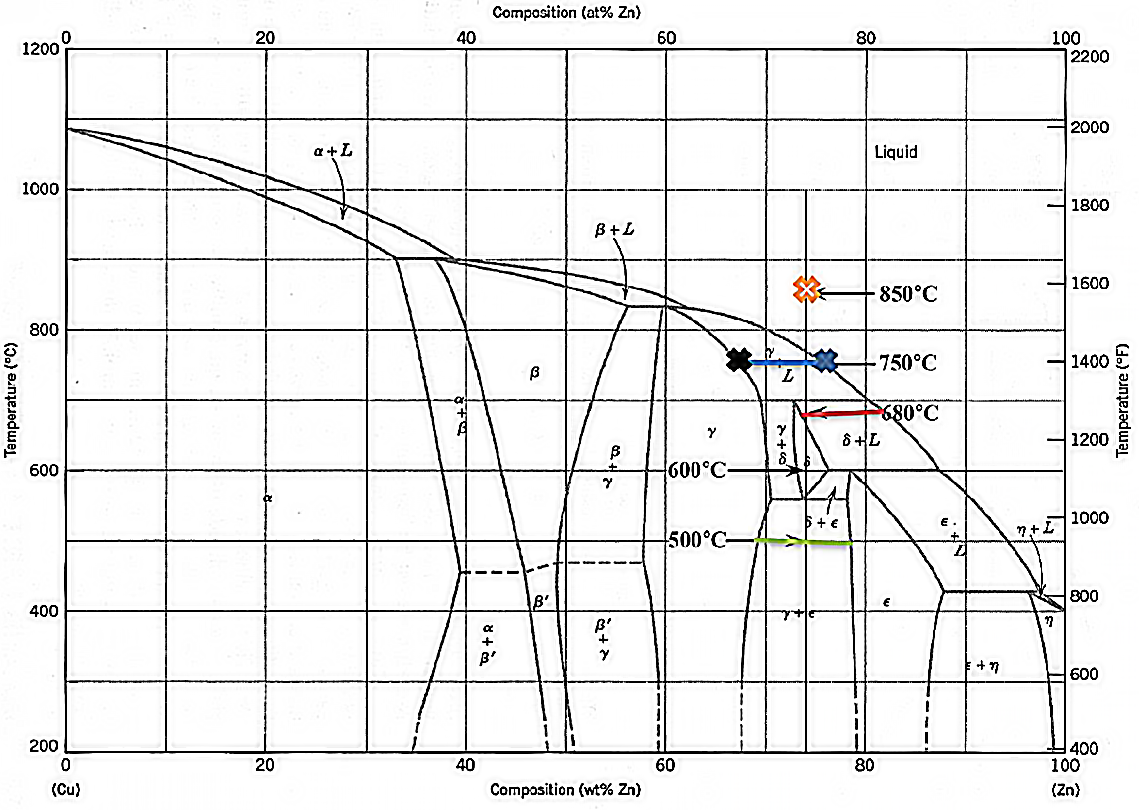At the temperature 850°C, the (L) liquid phase is witnessed. The Composition of the respective single-phase can be witnessed in the phase diagram.  CL = 74 wt % of Zn and 26 wt. % of Cu.

At the temperature 750°C, γ as well as L phases are witnessed.

At the temperature 680°C, δ as well as L phases are witnessed. The compositions of the two phases are noted from the tie-line at this respective temperature.

Composition of δ: Cδ = 73 wt % of Zn and 27 wt % of Cu
Composition of liquid (L): CL = 82 wt % of Zn and 18 wt % of Cu

At the temperature 600°C, from the diagram the phase δ is witnessed. composition of this particular phase is called C δ = 74 wt % of Zn-26 wt. % of Cu

At the temperature 500°C, both ε and γ phases can be witnessed; the compositions of these phases are determined from the GREEN tie-line at 500°C temperature.
C γ = 69 wt % of Zn-31 wt % of Cu; Cε = 78 wt. % of Zn-22 wt% of Cu